# HLOOKUP in Excel – With Examples

HLOOKUP function in Excel is a sibling of VLOOKUP function. The H in the HLOOKUP stands for “Horizontal” and hence it is often called as Horizontal Lookup.

HLOOKUP is a very useful function for creating horizontal lookups, but as most of the tables that we deal with are vertical hence this function is not very popular.

The task of HLOOKUP function is to search for a value in the topmost row of a table, and then return a corresponding value in the same column from a row you specify.

### Definition and Syntax of HLOOKUP Function in Excel:

Microsoft Excel defines HLOOKUP as a function that “looks for a value in the top row of a table or array of values and returns the value in the same column from a row you specify”.

### Syntax of Excel HLOOKUP:

The syntax of HLOOKUP function in Excel is as follows:

`=HLOOKUP(lookup_value, table_array, row_index_num, range_lookup)`

Here, ‘`lookup_value`’ refers to a value that is to be searched in the topmost row of the table. ‘`lookup_value`’ can be a value, a reference or a text string.

`table_array`’ is the range reference or range name of an array of values, inside which in which the data is to be looked up.

`row_index_num`’ is the row number in the ‘`table_array`’, from which the matching value is to be returned. A ‘`row_index_num`’ equal to 1 returns a value from the topmost row in the ‘`table_array`’ and similarly a ‘`row_index_num`’ equal to 2 returns a value from the second row of the ‘`table_array`’.

`range_lookup`’ argument accepts a Boolean value that specifies whether you want Horizontal Lookup function to return an exact match or an approximate match. TRUE stands for approximate match while FALSE stands for an exact match.

### How to Use HLOOKUP in Excel:

Now, let’s understand how to use this Function in Excel.

Consider, we have a Student Table as shown below: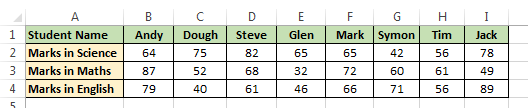Objective: In this case, our objective is to fetch Steve’s marks in English using Horizontal Lookup.

So, we will try to apply an HLOOKUP to get the result.‘lookup_value’: As we know that we have to find the marks of Steve, so our ‘`lookup_value`’ will be a “Steve”.‘table_array’: In this argument we give the reference of our table i.e. `A1:I4`.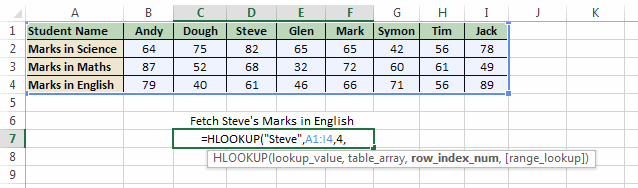‘row_index_num’: The ‘`row_index_num`’ in this case would be 4 as here we have to fetch a value from the fourth row of the table.‘range_lookup’:`range_lookup`’ will be FALSE as here we only want to fetch the exact match value.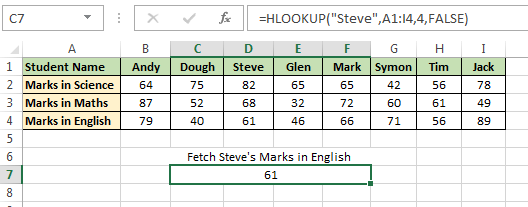The result of this formula is 61.

### Few important points about HLOOKUP:

• Horizontal Lookup performs a case insensitive lookup. This means, it treats “STEVE” and “steve” as same.
• While using HLOOKUP function ‘`lookup_value`’ should always be in the topmost row of the ‘`table_array`’.
• `range_lookup`’ is an optional argument. If it is omitted then HLOOKUP takes its default value as TRUE (approximate match).
• If HLOOKUP cannot find the ‘`lookup_value`’, and ‘`range_lookup`’ is TRUE (approximate match), it uses the largest value that is less than ‘`lookup_value`’.
• Similar to VLOOKUP, HLOOKUP also supports wildcard characters (like: ‘*’, ‘?’) in the ‘`lookup_value`’ argument (only if ‘`lookup_value`’ is text). [See Example 2]
• If ‘`range_lookup`’ is FALSE and HLOOKUP is unable to find the ‘`lookup_value`’ in the defined range, then it returns a #N/A error.
• If the ‘`row_index_num`’ is less than 1, HLOOKUP returns #VALUE! error. If it is greater than the number of columns in ‘`table_array`’, then it returns #REF! error.

### 5 Examples of Excel HLOOKUP:

Now, let’s see some examples of Horizontal Lookup Function.

Example 1: Using the below table, find the Marks in English of a student who has got 75 marks in Science.We can use this formula to get the result: `=HLOOKUP(75,B2:I4,3,FALSE)`This resultant of this formula is 40.

Explanation:

• The first argument to the function i.e. ‘`lookup_value`’ = 75 (Marks of the student in Science)
• Second argument i.e. ‘`table_array`’ = B2:I4 (Range of student table)
• Third argument i.e. ‘`row_index_num`’ = 3 (the row number whose value the HLOOKUP function should return)
• Fourth argument i.e. ‘`range_lookup`’ = FALSE (Signifies that we only want the exact match)

Example 2: Using the same table as above, write an Horizontal LookUp formula to find the Maths marks of a student whose name starts with ‘G’.

To do the we can use the formula: `=HLOOKUP("G*",A1:I4,3,FALSE)`

Note: In this example we have used a wild card character “*”.Explanation:

• The first argument to the function i.e. ‘`lookup_value`’ = “G*” (which signifies the name that  stats with ‘G’ character)
• Second argument i.e. ‘`table_array`’ = A1:I4 (Range of student table)
• Third argument i.e. ‘`row_index_num`’ = 3 (the row number to be returned)
• Fourth argument i.e. ‘`range_lookup`’ = FALSE (Signifies that we only want the exact match)

Example 3: Here in this example we have two tables as shown, now our task is to apply an HLOOKUP formula and populate the History marks in the first table.This can be done by using the formula: `=HLOOKUP(B1,\$M\$1:\$T\$2,2,FALSE)`

And then dragging it to all the columns using the fill handle.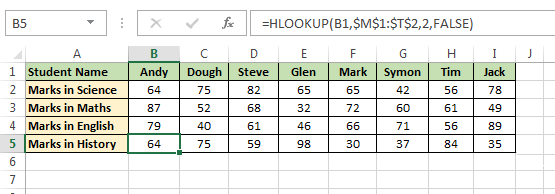Note: If you are wondering what these dollar signs ‘\$’ are doing in this formula, then I would suggest you to read this post.

Explanation:

• The first argument to the function i.e. ‘`lookup_value`’ = “B1” (which signifies the name of Student)
• Second argument i.e. ‘`table_array`’ = \$M\$1:\$T\$2 (Range of second student table)
• Third argument i.e. ‘`row_index_num`’ = 2 (the row number whose value the function should return)
• Fourth argument i.e. ‘`range_lookup`’ = FALSE (Signifies that we only want the exact match)

Example 4: In this example we have an Element Table as shown below and our task is to find the  Atomic Mass of Boron.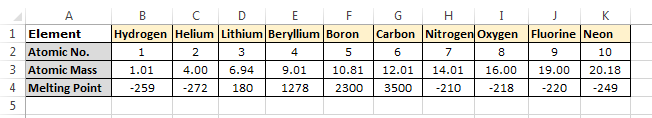To do this we can use the HLOOKUP as: `=HLOOKUP(F1,B1:K4,3,FALSE)`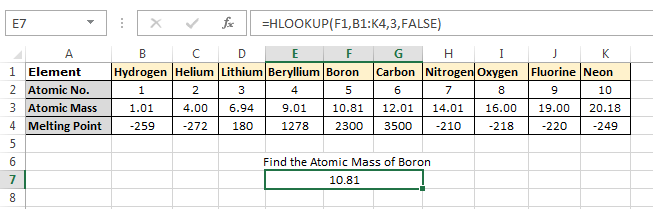Explanation:

• The first argument to the function i.e. ‘`lookup_value`’ = “F1” (which is the address of the cell containing Boron)
• Second argument i.e. ‘`table_array`’ = B1:K4 (Range of Element table)
• Third argument i.e. ‘`row_index_num`’ = 3 (the row number to be returned)
• Fourth argument i.e. ‘`range_lookup`’ = FALSE (Signifies that we only want the exact match)

Example 5: Using the above element table find the Melting Point of an element whose Atomic Mass is 15 or slightly less than it.

In this case we can use the formula: `=HLOOKUP(15,B3:K4,2,TRUE)`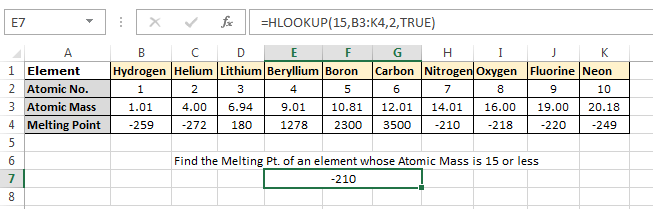Note: Notice in this example we have set the ‘`range_lookup`’ argument as TRUE, this is means that, if an exact match is not found, the next largest value that is less than ‘`lookup_value`’ is returned.

Explanation:

• The first argument to the function i.e. ‘`lookup_value`’ = “15” (it is the Atomic Mass to be searched)
• Second argument i.e. ‘`table_array`’ = B1:K4 (Range of Element table)
• Third argument i.e. ‘`row_index_num`’ = 2 (the row number to be returned)
• Fourth argument i.e. ‘`range_lookup`’ = TRUE (Signifies that we only want the exact or approximate match)

In this example, as you can see that we have set ‘`range_lookup`’ = TRUE, because none of the elements present in table have Atomic Mass equal to 15. Hence, when HLOOKUP is unable to find any element the Atomic Mass 15 it picks up the nearest (but smaller than ‘`lookup_value`’) number i.e. 14.01 and returns its corresponding Meting point. And, hence the result is -210.

### How to use HLOOKUP in VBA:

Using HLOOKUP in VBA is very easy. For using HLOOKUP in VBA you simply need to remember that you can find it under “`Application.WorksheetFunction`”.

Example 6: Write a VBA program using HLOOKUP, to find the marks of the specified student in all the subjects from the below table.Below is the code to this:

`Sub H_LOOKUP()On Error GoTo ErrorHandlerDim student As StringDim Result As Stringstudent = InputBox("Enter the student Name:")If Len(student) > 0 Then Result = "Science - " & Application.WorksheetFunction.HLookup(student, ActiveSheet.Range("A1:I5"), 2, False) Result = Result & vbNewLine & "Maths - " & Application.WorksheetFunction.HLookup(student, ActiveSheet.Range("A1:I5"), 3, False) Result = Result & vbNewLine & "English - " & Application.WorksheetFunction.HLookup(student, ActiveSheet.Range("A1:I5"), 4, False) Result = Result & vbNewLine & "History - " & Application.WorksheetFunction.HLookup(student, ActiveSheet.Range("A1:I5"), 5, False) MsgBox student & " has got following Marks:" & vbNewLine & ResultEnd IfExit SubErrorHandler:If Err.Number = 1004 Then MsgBox "Student Not found in the records!"Else MsgBox "Some Error Occurred"End IfEnd Sub`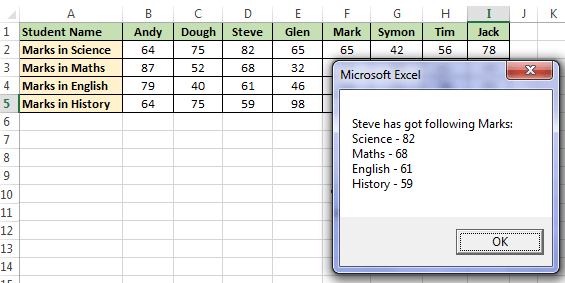Explanation:

In this code we are using multiple Horizontal LookUp formulas to fetch the marks of the student in different subjects. If the student entered by the user is not in the table, the code pops out a message saying “Student Not found in the records!”

Recommended Reading : MsgBox, InputBox, Concatenate In Excel, If Function, On Error Statement, VLOOKUP in VBA

### Using ISNA Function with HLOOKUP:

As I have already told, the HLOOKUP function throws a #N/A error if ‘`range_lookup`’ is FALSE and HLOOKUP is unable to find the ‘`lookup_value`’ in the defined range.

These #N/A errors do not look good and hence it is better to hide them and display some meaningful message.

Example 7: In this example we will see how to hide #N/A errors. Here, we will try to find the Melting Point of an element whose Atomic Mass is 11.

So, we will write a formula as: `=HLOOKUP(11,B2:K4,3,FALSE)`Now, as this #N/A error looks ugly so we will try to use the HLOOKUP with ISNA function to display a meaningful message.

The resultant formula will become: `=IF(ISNA(HLOOKUP(11,B2:K4,3,FALSE))=TRUE, "Value Not Found", HLOOKUP(11,B2:K4,3,FALSE))`ISNA function returns TRUE if there is a #N/A error in the formula.

### How to return multiple values from a single Horizontal LookUp:

Until now we have seen that HLOOKUP only returns a single value. But you can create an array HLOOKUP function to fetch multiple records.

Let’s make it clearer by understanding this with an example.

Example 8: Let’s consider, we have a table as shown below. Now, in this table we want to fetch the Marks of Glen in all the subjects.So, we will use a formula as: `=HLOOKUP("Glen",B1:I5,{1,2,3,4,5},FALSE)`

Please note that this is an array formula. To enter this formula, select the number of cells equal to the number of rows that you want HLOOKUP to return.

With all the cells selected enter the formula bar, paste the above formula and press Ctrl + Shift + Enter. Pressing Ctrl + Shift + Enter will enclose the above formula in curly brackets like:

`{=HLOOKUP("Glen",B1:I5,{1,2,3,4,5},FALSE)}`

And the result will be displayed.In, the above image see how a single HLOOKUP returns all the Marks of Glen.

If you still didn’t get it, then see the below animated image: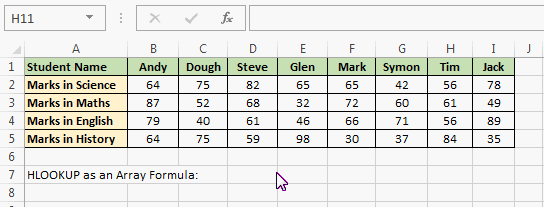So, this was all about HLOOKUP in Excel. Download the spreadsheet containing all the above examples here. And do share your views and queries related to the topic.About Ankit Kaul

Ankit is the founder of Excel Trick. He is tech Geek who loves to sit in front of his square headed girlfriend (his PC) all day long. :D. Ankit has a strong passion for learning Microsoft Excel. His only aim is to turn you guys into 'Excel Geeks'.

1.Rahim Zulfiqar Alisays

Thanks this is really cool and awesome,
Can you please share the XLSX file.

•Ankit Kaulsays

•Rahim Zulfiqar Alisays

Thanks 🙂

•Ankit Kaulsays

My Pleasure 🙂

2.amitsays

Thanks friend
Now I want know about macro so can u give me some like this note with example ?
•Ankit Kaulsays